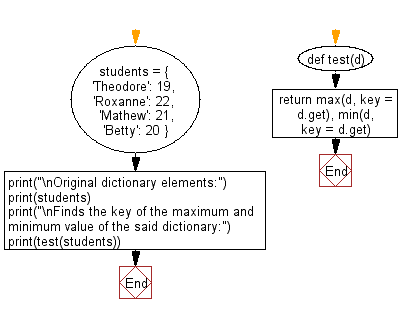﻿ Python: Find the key of the maximum, minimum value in a dictionary - w3resource# Python: Find the key of the maximum, minimum value in a dictionary

## Python dictionary: Exercise-80 with Solution

Write a Python program to find the key of the maximum value in a dictionary.

• Use max() with the key parameter set to dict.get() to find and return the key of the maximum value in the given dictionary.
• Use min() with the key parameter set to dict.get() to find and return the key of the minimum value in the given dictionary.

Sample Solution:

Python Code:

``````def test(d):
return max(d, key = d.get), min(d, key = d.get)

students = {
'Theodore': 19,
'Roxanne': 22,
'Mathew': 21,
'Betty': 20
}
print("\nOriginal dictionary elements:")
print(students)
print("\nFinds the key of the maximum and minimum value of the said dictionary:")
print(test(students))
```
```

Sample Output:

```Original dictionary elements:
{'Theodore': 19, 'Roxanne': 22, 'Mathew': 21, 'Betty': 20}

Finds the key of the maximum and minimum value of the said dictionary:
('Roxanne', 'Theodore')
```

Flowchart:## Visualize Python code execution:

The following tool visualize what the computer is doing step-by-step as it executes the said program:

Python Code Editor:

Have another way to solve this solution? Contribute your code (and comments) through Disqus.

What is the difficulty level of this exercise?

Test your Programming skills with w3resource's quiz.

﻿

## Python: Tips of the Day

Decapitalizes the first letter of a string:

Example:

```def tips_decapitalize(s, upper_rest=False):
return s[:1].lower() + (s[1:].upper() if upper_rest else s[1:])
print(tips_decapitalize('PythonTips'))
print(tips_decapitalize('PythonTips', True))
```

Output:

```pythonTips
pYTHONTIPS
```